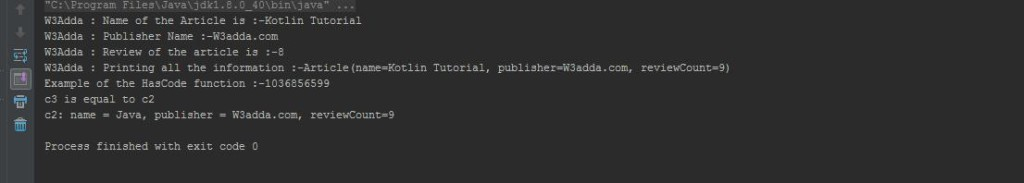# Kotlin Data Class

In this tutorial you will learn about the Kotlin Data Class and its application with practical example.

## Kotlin Data Class

The class which is declared as “data” known as data class. All data classes must have at least one primary constructor and primary constructor should have at least one parameter. It can not be open, abstract, inner and sealed. Data class can extend other classes also can implement interface. It have some inbuilt function like “toString()” ,”hashCode(),equals() ,copy() etc. and we can use them.

Example: Let’s get understand it by an example. See below code.

Here, I have used some inbuilt function which are created by the compiler, when we declared class as “data” class. Let’s understand inbuilt function one by one

toString(): This function is used to returns a string representation of the object. Check below code which is used in above example.

hashCode(): This function is used to returns hash code of the object. Check below lines which is used in above example for printing hashCode of object.

Copy(): This function is used to create a copy of an object with some of its properties and also can copy whole object. Check below lines.

Equals(): This function is used to check whether objects are equals. Check below lines.

Output: Let’s run this code you will get output like below image.In this tutorial we have learn about the Kotlin Data Class and its application with practical example. I hope you will like this tutorial.